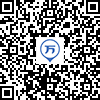120:002012年海南省公务员考试《行测》真题

1
(单项选择题)

2011年我国改革开放和社会主义现代化建设取得新的重大成就，其中包括（　）。

• A.

人均GDP超过3000美元

• B.

外汇储备首次超过日本居世界第一

• C.

城镇化率超过50%

• D.

粮食总产量首次超过10万亿斤

• A
• B
• C
• D

2
(单项选择题)

• A.

齐国的纬度最高

• B.

楚国的纬度最低

• C.

燕国的经度最小

• D.

秦国的经度最大

• A
• B
• C
• D

3
(单项选择题)

• A.

羌笛何须怨杨柳，春风不度玉门关。

• B.

渭城朝雨浥轻尘，客舍青青柳色新。

• C.

今宵酒醒何处，杨柳岸，晓风残月。

• D.

庭院深深深几许，杨柳堆烟，帘幕无重数。

• A
• B
• C
• D

4
(单项选择题)

• A.

《西厢记》—《汉宫秋》—《桃花扇》—《牡丹亭》

• B.

《西厢记》—《桃花扇》—《汉宫秋》—《牡丹亭》

• C.

《汉宫秋》—《桃花扇》—《牡丹亭》—《西厢记》

• D.

《汉宫秋》—《牡丹亭》—《桃花扇》—《西厢记》

• A
• B
• C
• D

5
(单项选择题)

• A.

燕子丹与荆轲分坐八仙桌两侧，秉烛夜谈，谋划赴咸阳刺杀秦王计划

• B.

毛泽东与李宗仁幽默地说：德邻先生，你这次归国，是误上了贼船啊

• C.

魏征劝谏李世民时，说道：陛下当先天下之忧而忧，后天下之乐而乐

• D.

越王勾践兵败后给吴王夫差当奴仆，以红薯充饥，每晚则睡在柴垛上

• A
• B
• C
• D

6
(单项选择题)

• A.

士别三日，当刮目相看——用发展的眼光看问题

• B.

草船借箭——人可以认识并利用规律

• C.

张飞醉酒失徐州，借酒破张郃——矛盾是对立统一的

• D.

望梅止渴——理性认识依赖于感性认识

• A
• B
• C
• D

7
(单项选择题)

①邓小平访美

②中美发布上海联合公报

③尼克松总统访华

④福特总统访华

• A.

②③①④

• B.

②①④③

• C.

①②③④

• D.

③②④①

• A
• B
• C
• D

8
(单项选择题)

• A.

上合组织

• B.

东盟十国

• C.

金砖国家

• D.

G20

• A
• B
• C
• D

9
(单项选择题)

• A.

组织社区志愿者开展邻里互动活动

• B.

提高临床一线医护人员的待遇水平

• C.

通过微博向群众提供政务服务资讯

• D.

设奖鼓励市民投诉举报食品安全问题

• A
• B
• C
• D

10
(单项选择题)

• A.

社会主义首先要发展生产力

• B.

社会主义也可以搞市场经济

• C.

中国要警惕右，但主要是防止“左”

• D.

搞建设至少不比搞革命容易

• A
• B
• C
• D

11
(单项选择题)

• A.

村委会规定村里的荒山不得以招标、拍卖等形式实行承包经营

• B.

村民乙外出经商，村委会不同意乙在承包期内放弃承包权

• C.

村民丙全家转为城市户口，村委会取消丙承包期内的承包权

• D.

村委会规定凡是嫁往外地的本村妇女，承包地应一律归还村委会

• A
• B
• C
• D

12
(单项选择题)

• A.

某男擅自按市价出售双方共有住房，其妻要求追回

• B.

某女起诉请求离婚，要求分割其丈夫的工伤补助金

• C.

按父母遗嘱某女继承全部家产，其兄要求平分家产

• D.

某夫妻已育有一个健康男孩，现申请收养一名孤儿

• A
• B
• C
• D

13
(单项选择题)

• A.

未经业主委员会同意，顶楼业主不得私自封闭屋顶天台

• B.

经物业管理处同意，业主可在自家临街外墙设置广告牌

• C.

未经业主委员会同意，开发商无权出售架空层内的车库

• D.

经工商行政管理部门许可，业主可将自家住宅改为商店

• A
• B
• C
• D

14
(单项选择题)

• A.

某企业当年生产的库存品

• B.

某人购买的一套二手房

• C.

某人持有国债的利息收入

• D.

某人在家从事家务劳动

• A
• B
• C
• D

15
(单项选择题)

• A.

以美元计价的大宗商品价格上涨

• B.

世界新兴经济体将增加美元储备

• C.

我国产品在美国市场竞争力上升

• D.

我国经销商将减少进口美国产品

• A
• B
• C
• D

16
(单项选择题)

2011年12月中央经济工作会议提出“牢牢把握发展实体经济这一坚实基础”。下列产业中属于实体经济的是（　）。

①房地产业②仓储服务业③旅游产业④收藏业⑤电信产业

• A.

③⑤

• B.

②③⑤

• C.

①②③⑤

• D.

①②③④⑤

• A
• B
• C
• D

17
(单项选择题)

• A.

我国实行义务兵与志愿兵相结合、民兵与预备役相结合的兵役制度

• B.

普通高等学校毕业生征集服现役的年龄可以放宽至二十四周岁

• C.

义务兵服现役的期限为三年，士官服现役的期限一般不超过三十年

• D.

士兵预备役的年龄为十八周岁至三十五周岁，根据需要可适当延长

• A
• B
• C
• D

18
(单项选择题)

• A.

在全国范围内免征农业税

• B.

在全国范围内实行粮食直补

• C.

实施农业科技入户工程

• D.

实施最严格的耕地保护制度

• A
• B
• C
• D

19
(单项选择题)

• A.

东风是个精，不下也要阴

• B.

天上鱼鳞云，地下雨淋淋

• C.

朝霞不出门，晚霞行千里

• D.

百日连阴雨，总有一日晴

• A
• B
• C
• D

20
(单项选择题)

• A.

雨水、谷雨反映降水现象

• B.

立春、春分反映季节变化

• C.

惊蛰、清明反映自然物候现象

• D.

小满、芒种与农作物成熟和收成相关

• A
• B
• C
• D